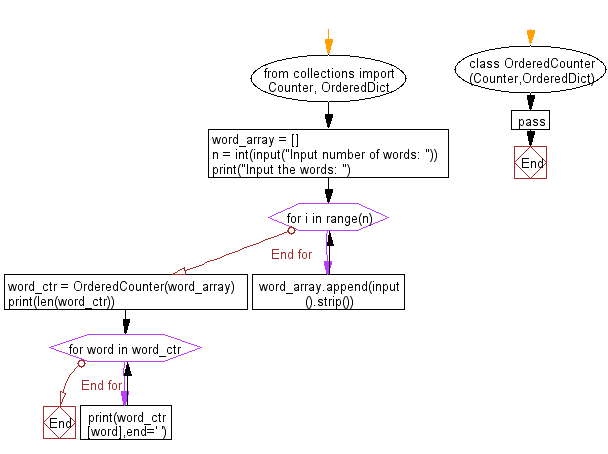﻿ Python: Accept some words and count the number of distinct words - w3resource# Python: Accept some words and count the number of distinct words

## Python Collections: Exercise-5 with Solution

Write a Python program that accept some words and count the number of distinct words. Print the number of distinct words and number of occurrences for each distinct word according to their appearance.

Sample Solution:

Python Code:

``````from collections import Counter, OrderedDict
class OrderedCounter(Counter,OrderedDict):
pass
word_array = []
n = int(input("Input number of words: "))
print("Input the words: ")
for i in range(n):
word_array.append(input().strip())
word_ctr = OrderedCounter(word_array)
print(len(word_ctr))
for word in word_ctr:
print(word_ctr[word],end=' ')
```
```

Sample Output:

```Input number of words:  5
Input the words:
Red
Green
Blue
Black
White
5
1 1 1 1 1
```

Flowchart:## Visualize Python code execution:

The following tool visualize what the computer is doing step-by-step as it executes the said program:

Python Code Editor:

Have another way to solve this solution? Contribute your code (and comments) through Disqus.

What is the difficulty level of this exercise?

Test your Programming skills with w3resource's quiz.

﻿

## Python: Tips of the Day

Use Reversed() In for Loops:

```>>> tasks = ['laundry', 'picking up kids', 'gardening', 'cooking']